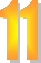#### You may also like### DOTS Division

Take any pair of two digit numbers x=ab and y=cd where, without loss of generality, ab > cd . Form two 4 digit numbers r=abcd and s=cdab and calculate: {r^2 - s^2} /{x^2 - y^2}.### Latin Numbers

Can you create a Latin Square from multiples of a six digit number?### 2-digit Square

A 2-Digit number is squared. When this 2-digit number is reversed and squared, the difference between the squares is also a square. What is the 2-digit number?

# Novemberish

##### Age 14 to 16Challenge Level(a) A four digit number (in base $10$) $aabb$ is a perfect square. Discuss ways of systematically finding this number.
(b) Prove that $11^{10}-1$ is divisible by $100$.